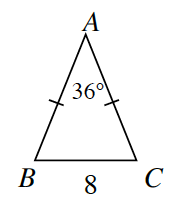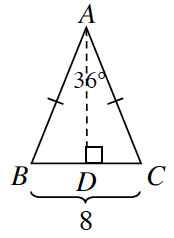### Home > INT2 > Chapter 8 > Lesson 8.1.1 > Problem8-13

8-13.Tromika wants to calculate the area of the isosceles triangle at right.

1. She decides to start by drawing a height from vertex $A$ to side $\overline{BC}$ as shown below. Will the two smaller triangles be congruent? In other words, is $∆ABD ≅ ∆ACD$? Why or why not?Look at the two triangles that are created. They share a side, and the tick marks mean the side lengths are equal. Are the triangles congruent?

Yes, they are congruent by $\text{HL}≅$.

2. What is $m∠DAB$? $BD$?

$∠DAB≅∠DAC$ since congruent triangles have congruent parts.

$m∠DAB=18^{\circ}$$BD = 4$

3. Determine $AD$. Show how you got your answer.

Which trig ratio can you use to determine this length?

4. Calculate the area of $∆ABC$.

How do you find the area of a triangle? Do you have enough information to calculate the area?